# Three dice

What is the probability that when we roll three dice, we roll the numbers 1,2,3?

p =  0.463 %

### Step-by-step explanation: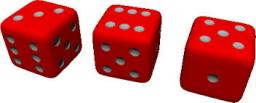Did you find an error or inaccuracy? Feel free to write us. Thank you!Tips to related online calculators
Would you like to compute count of combinations?

## Related math problems and questions:

• DicesWe will throw two dice. What is the probability that the ratio between numbers on first and second dice will be 1:2?
• One threeWe throw two dice. What is the probability that max one three falls?
• Two diceWe roll two dice. What is the probability that the sum of the falling numbers is greater than 3?
• We rollWe roll two dice A. - what is the probability that the sum of the falling numbers is at most 4 B. - is at least 10 C. - is divisible by 5?
• Roll the diceWhat is the probability that if we roll the dice, a number less than 5 falls?
• The diceWhat is the probability of events that if we throw a dice is rolled less than 6?
• Balls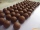From the bag with numbered balls (numbers 1,2,3,. ..20) we pick one ball. What is the probability of choosing a number containing 1?
• ThrowWe throw 2 times with 2 dices. What is the probability that the first roll will fall more than sum of 9 and the second throw have sum 3 or does not have the sum 4?
• Six on diceWhat is the probability that when throwing two dice will fall at least one six?
• Sum 10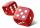What is the probability that two dice throw twice in a row will result the sum of 10?
• Perfect cubesSuppose a number is chosen at random from the set (0,1,2,3,. .. ,202). What is the probability that the number is a perfect cube?
• One dice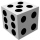Calculate the probability of one dice roll with the numbers 1, 2, 3, 4, 5, 6 on the walls. Write the results in a notebook in the shape of a fraction in the basic form: 2/3. a, The number 1 falls on the cube. b, The number 5 falls on the cube. c, An even
• Sum on diceWe have two dice. What is the greater likelihood of fall a total sum 7 or 8 ? (write 7, 8 or 0 if the probabilities are the same)?
• Drawing from a hatWhen drawing numbers from a hat from 1 to 35, we select random given numbers. What is the probability that the drawn numbers will be divisible by 8 and 2?
• Sum of fall dices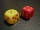What is the probability that the sum of 9 will fall on a roll of two dice? Hint: write down all the pairs that can occur as follows: 11 12 13 14 15. . 21 22 23 24. .. . 31 32. .. . . . . . .. . 66, count them, it's the variable n variable m: 36, 63,. .. .
• Air draftThe numbers 1,2,3,4,5 are written on five tickets on the table. Air draft randomly shuffled the tickets and composed a 5-digit number from them. What is the probability that he passed: and, the largest possible number b, the smallest possible number c, a
• Probability - tickets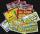What is the probability when you have 25 tickets in 5000 that you not wins the first (one) prize?